The Last Challenge of Modern Physics | OMICS International
Journal of Physical Mathematics

# The Last Challenge of Modern Physics

Michaud A*

SRP Inc Educational Research Service Quebec, Canada

*Corresponding Author:
Michaud A
SRP Inc Educational Research Service
Tel: 416-555-0171
E-mail: [email protected]

Received Date: February 22, 2017; Accepted Date: March 20, 2017; Published Date: March 22, 2017

Citation: Michaud A (2017) The Last Challenge of Modern Physics. J Phys Math 8:217. doi: 10.4172/2090-0902.1000217

Copyright: © 2017 Michaud A. This is an open-access article distributed under the terms of the Creative Commons Attribution License, which permits unrestricted use, distribution, and reproduction in any medium, provided the original author and source are credited.

Visit for more related articles at Journal of Physical Mathematics

#### Abstract

Synthesis of the current state of research on the conversion processes involving electromagnetic energy and mass, and description of an expanded space geometry that may help resolve many of the remaining issues

#### Keywords

Hamiltonian; Trispatial geometry; Trispatial LC equations; Quantum of action; Quantum of induction; Adiabatic energy induction; 3-Spaces geometry

#### Objective Physical Reality

Over the course of the 20th century, a whole bunch of particles has been identified, or "defined", that have mostly been categorized as part of the Standard Model of particle physics. The Standard Model is the superset of all particles that are deemed to exist and serve as material for the construction of the material universe, which is the foundation of objective physical reality.

They can be regrouped into numerous subsets: virtual particles, unstable complex particles, unstable elementary particles, stable complex particles, stable elementary particles, and finally, neutrinos. We will examine each of these subsets at the general level.

But before proceeding, let us examine the tools that we have at our disposal to identify and verify the physical existence of these elementary particles.

#### Destructive vs. Non-Destructive Scattering

Verifying the existence of elementary particles can be achieved only by colliding them with each other. Their trajectories, deflected during such encounters, can be recorded by various means to be subsequently studied and interpreted. In fact, the recorded trace of the deflected trajectories of colliding particles is the only proof out of any doubt of the physical existence of these particles.

Elementary particle do not interact during such collisions like hard solid objects as could be expected from our macroscopic perspective, but like elastic "objects" due to their common electromagnetic nature. They can interact either electrically according to the well known inverse square law of distance between such particles, or magnetically according to the less familiar inverse cube magnetic interaction law between the same particles . From our macroscopic perspective, they behave as if they electrically "attracted" or "repelled" each other according to the inverse square law.

The closer they come to each other, the more strongly they seem to electrically repel each other if their electric charges are of the same sign, and the more strongly they seem to electrically attract each other if they have opposite electric signs. Similarly, the closer they come to each other, the more strongly they will magnetically repel each other if they interact in parallel spin alignment, and the more strongly they will magnetically attract each other if they interact in anti-parallel spin alignment.

Exploratory high energy collisions of fundamental particles can be carried out in two different ways, which are the non-destructive collision mode or the destructive collision mode, and the absence of a clear description in textbooks of the difference between both methods has been the source of widespread confusion.

#### Non-Destructive Scattering

Non-destructive scattering was used for a short period of time during the second half of the 1960s to explore the only two stable composite particles in existence, the proton and the neutron, which also are the only components of all existing atomic nuclei. Since it had previously been confirmed that they occupy a measurable volume in space, this hinted at the possibility that they could have an internal structure involving smaller particles, and consequently, that they might not be elementary. The proton was discovered in 1919 by Ernest Rutherford and the neutron in 1932 by James Chadwick.

Non-destructive collisions with neutrons and protons (nuclei of hydrogen and deuterium atoms captive in water molecules, for example) involves colliding them with electrons or positrons sufficiently accelerated with magnets thus, increasing their momentum sustaining kinetic energy, to enter the nucleon structures, but with insufficient energy to knock the components making up their internal structure out of their structure.

Contrary to protons and neutrons, electrons and positrons seem not to occupy any measurable volume in space and always behave as if they were point-like in the mathematical sense each time they are involved in collisions. They are considered "elementary" because the most energetic non-destructive head-on collisions between 2 electrons are, for example, the closer they come to each other's "point-like center" before rebounding, without any unbreachable limit at some distance from this center having been reached. They were then the ideal projectiles to attempt resolving the enigma of the internal structure of protons and neutrons. The electron was discovered in 1856 by Joseph Thompson and the positron in 1932 by Carl Anderson.

Electrons or positrons that met no obstacle inside nucleons crossed completely through the volume occupied by nucleons, but had their trajectories deflected to various degrees depending on how close they came to the inner components of nucleons, which were thus directly detected for the first time. Some of these incident electrons or positrons were very strongly deflected, some even directly backscattered when their trajectories happened to be in direct line with one of these inner components.

Careful analysis revealed that these inner components are electrically charged like electrons and positrons, because their deflected trajectories all obeyed the same deflection law that governs collisions between two electrons or two positrons, that is, the inverse square Coulomb law .

The closer the incident particles came to these inner components during these flybys, the more strongly their trajectories were deflected. The incident negative electrons were attracted to the positively charged inner components and repelled by the negatively charged inner components, while positive positrons were attracted to the negatively charge inner components and repelled by the positively charged inner components. On final analysis, the scattering patterns revealed by the deflected trajectories led to the confirmed discovery that only two different scatterable elementary particles charged in opposition existed inside protons and neutrons.

The positive component was named up quark, possessing 2/3 of the charge of the positron, and the negative component was named down quark, possessing 1/3 of the charge of the electron . This is how the discovery was made that the inner scatterable structure of the proton is made of 2 up quarks and 1 down quark (uud), while that of the neutron is made of 1 up quark and 2 down quarks (udd).

It was discovered furthermore, that the up quark was only marginally more massive than the electron and that the down quark was only marginally more massive than the up quark (, p. 11-6). Let us also note that the addition of the fractional charges of these inner elementary particles directly explains the measurable electric charges of the proton and the neutron: +2/3 +2/3 -1/3=+1 for the proton and 2/3 -1/3 -1/3=0 for the neutron.

#### Destructive Scattering

Finding no other scatterable components inside nucleons, destructive scattering started being used towards the end of the 1960s, and has been used to higher and higher energy levels ever since. This method may involve the liberation of the carrying energy of two same sign particles, such as two electrons, that occurs when two such particles collide head-on against each other, causing this carrying energy to escape as highly energetic bremmsstrahlung photons as their motion is brutally stopped momentarily, or the physical destruction of two opposite sign elementary particles, such as an electron and a positron, when these particles ultimately meet, which causes the energy of which their rest masses are made to convert to free energy (electromagnetic photons), thus causing them to cease existing under their initial form, on top of their carrying energy also being released as electromagnetic photons.

When the destructive level is reached during such collisions, huge amounts of free electromagnetic energy are liberated as the incident bullet (an electron, for example) and the up or down quark that it directly collides against are converted to energy. The total amount of liberated energy is made up of all of the kinetic energy sustaining the momentum of the incoming electron, plus all of the adiabatic energy of the quark being scattered against , plus the energy that made up the rest masses of the quark and of the electron involved if they also convert.

Whenever such a huge amount of free electromagnetic energy is liberated, it immediately recongeals back into all sorts of transient metastable hyper-excited massive particles that are generically named "partons". The larger the amount of energy liberated during one such collision, the more massive the transient particles momentarily created will be, generally way more massive than the colliding particles.

During such destructive collisions, there may also be cases when the up or down quark involved will be ejected without having been destroyed, that is, without being converted to energy. Let us note that this barely diminishes the total amount of energy liberated, since the energy making up the rest masses of both types of quarks and of the incident electron or positron is very small with respect to that sustaining the momentum of the incoming particle and the stabilized adiabatic energy induced in the quark concerned at the moment of impact .

Note that the momentum sustaining energy of the incoming particle and the stabilized adiabatic energy induced in each up and down quark that are captive of the inner structure of nucleons, is kinetic energy in excess of their invariant rest mass energy, and unless specific identification is required, both types will generally be referred to in this text as "carrying energy" or "carrier-photon", for reasons that will become clear further on.

It is a fact that up and down quarks have never been observed moving freely after having been ejected while still displaying the same characteristics that they have when inside nucleons. This doesn't mean however that they have not been observed displaying different characteristics after having been ejected, which is an as of yet unexplored possibility that may well have prevented experimentalists from recognizing them as the same particle.

For example, if up and down quarks were in reality positrons and electrons whose masses and charge characteristics had been warped into these altered states by the stresses imposed by these most energetic least action equilibrium states that electrons and positrons could reach if they were the actual material that Nature used to build nucleons , then as one of them is scattered out of a nucleus without being destroyed, it would of course recover its normal electron or positron characteristics as soon as it escapes these warping stresses, which might directly explain why free moving up and down quarks have never been observed.

All partons produced during destructive collisions almost instantly decay into a cascade of transient states, the last stage of which always is one or other or a combination of the stable particles set, that is, electron, positron, proton, neutron and residual photons. All of these decay sequences have been thoroughly analyzed and are available in reference .

The more energetic the incident electron or positron will be, the more energy will be liberated as it destructively collides with one of the up or down quarks inside a nucleon, allowing more and more massive metastable partons to fleetingly appear before almost instantly decaying as previously described.

Even the recently much hyped about Higgs boson belongs to this parton category, actually the most massive parton yet detected as an up or down quark in an incident proton directly and destructively scattered against one of the up or down quarks of another proton at the LHC facility.

Four of the first partons that were long-lived enough to be detected in the 1970s were given the names charm quark, strange quark, bottom quark and top quark, because they seemed to satisfy the most popular theory of the time, even though, like all other partons, they all almost immediately decay into one or other of the stable particles subset.

Unfortunately, all of these short-lived partons are useless as far as describing normal matter in the universe is concerned, because they can exist only outside of protons and neutrons since they are created by means of this type of destructive scattering. Under no circumstance could they ever be identified within proton or neutron structures via non-destructive scattering.

This verified fact did not prevent the physics community from classifying these short-lived metastable massive states as part of the Standard Model, in an apparent endless search for more and more of these transient massive energy states, even though they obviously cannot be part of the stable material structures in the universe.

The same restriction applies to the variety of "virtual" particles that were "defined" such as gluons and "virtual photons" for example, which are mathematical concepts conjured up as mathematical artifacts to make the mathematical description of particles' interactions easier in currently popular theories.

A clear distinction must also be made between real electromagnetic photons, that are scatterable against electrons, and whose trajectories can be deflected by gravity , and Quantum Electrodynamics "virtual photons" which are mathematical metaphors conceived of by Richard Feynman  to make easier the calculation of interactions between elementary particles.

Moreover, the QED virtual photon metaphor unfortunately bundles together two fundamentally very different aspects of the relation between particles, that is, the Coulomb force proper and the "motion sustaining" or "momentum sustaining" kinetic energy induced by this force, which, when combined with the presence of the word "photon", induces a high level of confusion with "real electromagnetic photons" that are made of only free moving kinetic energy, as analyzed in reference , and as will be put in perspective further on.

In Nature, unstable partons also occur as fleeting massive states such as the various configurations of π and K mesons as well as hyperons, the latter being unstable complex particles still more massive than protons and neutrons, and as a few elementary unstable particles such as muon and tau, that have life expectancies never exceeding a few fractions of a second.

They are created as fleeting byproducts of cosmic radiation colliding with the nuclei of atoms in celestial bodies or their atmosphere, or as by-products of high energy particles' interaction in stars' coronas [8,9] and inside the permanently exploding star masses .

Let us note here that what has come to be generically named "cosmic radiation" is mostly made up of protons that are many orders of magnitude more energetic than can be achieved even in the LHC accelerator, which means that they can theoretically create fleeting partons more massive yet than the recently detected Higgs boson when they collide with other particles.

Just as in high energy accelerators, the final end product of the practically instantaneous decay of these naturally occurring partons is always a stable particle belonging to the stable massive particles subset already mentioned, besides photons and neutrinos.

The positron, known to be the anti-particle of the electron, is totally identical to the electron except for the sign of its charge , but does not become part of stable atoms contrary to the electron because it readily reverts to various electromagnetic photon states as soon as it individually interacts with an electron, also converting the electron to electromagnetic photon energy in this process which is named positronium decay.

Positrons being the anti-particles of electrons, they are viewed in the physics community as "antimatter" with respect to electrons, which are thus viewed as "normal" matter. There is incidentally a century old assumption that the universe is made almost entirely of "normal matter" (a concept that also includes protons and neutrons), and endless speculation is still ongoing as to why so few "antimatter" is to be found, which is deemed to directly contradict the principle of symmetry.

This issue may be completely resolved simply by considering that when the three charged scatterable elementary components of protons and neutrons are taken into account instead of the protons and neutrons themselves, which are not elementary, there exists by structure in the universe the exact same amount of normal matter and anti-matter, that is, the same number of negatively charged elementary particles and positively charged elementary particles [9,12,13].

Let us now examine the various particles subsets.

#### Virtual Particles

We can include in this subset "virtual photons", which are a mathematical metaphor that Feynman proposed in 1949  to introduce the notion of quantization of the interaction between charged particles, that allowed using the simpler static Lagrangian calculation method instead of the more elaborate Hamiltonian method to account for interactions between elementary charged particles. These virtual photons regroup in a single concept the Coulomb force and the intensity of the amount of related energy, a method easier to mathematically manipulate than the Hamiltonian which on its part more readily accounts for the infinitesimally progressive nature of energy variation.

Let us also include here gluons, which also are pseudo-quantized mathematical metaphors, but this time, of the presumably also progressive, but still not fully explored interaction at play between the charged inner components of nucleons in the frame of Quantum Chromodynamics; a yet to be explored interaction, one of whose laws can only be the Coulomb interaction, since up and down quarks are electrically charged.

What allows us to clearly distinguish these metaphorical virtual particles from real particles is the fact that it is impossible to prove their physical existence by means of the only available method, which is by colliding them directly with particles of the stable set.

In other words, all virtual particles turn out to be, without exception, simple mathematical concepts.

#### Unstable Complex Particles

Here we find various configurations of π and K mesons as well as hyperons, and the Higgs boson which are unstable complex particles still more massive than protons and neutrons, with life expectancy never exceeding a few fractions of a second.

What is remarkable about all unstable complex particles, which all are partons produced only in high energy accelerators, or as fleeting by-products of cosmic radiation, is that without exception as already mentioned, the final end product of their decay is systematically one or other, or a combination of the only known stable particles subset, that is, electrons, positrons, protons, neutrons and photons.

Consequently, these unstable complex particles could all be considered as only temporary hyper-energetic metastable states of the fundamental stable particles subset.

#### Unstable Elementary Particles

Here we find the various quarks, except the up and down quarks of course, and also all elementary partons, which, as already discussed quickly decay to end up as one or other particle of the stable particles subset.

In this category, we also find the muon particle, which is a second generation parton since it is typically generated from meson decay, which are first generation partons, and the tau particle, which is a first generation parton produced by destructive head-on electron-positron collisions, first observed at the SLAC facility in the 1970s. Both particles always leave behind a single electron as a solitary massive by product of their decay, besides neutrinos and occasionally a few gamma photons.

In a certain way, both particles muon and tau can be considered temporary unstable hyper-massive states of electrons that quickly decay to ultimate electron stable rest mass state by neutrino pairs emissions. The mechanics of electronic, muonic and tauic neutrino pair’s emission in the trispatial space geometry that soon will be described is analyzed in reference .

Of course, anti-muon and anti-tau leave behind a solitary positron instead of an electron.

#### Stable Complex Particles

In this category, we find only the proton, which is totally stable, and the neutron, that becomes totally stable when associated to protons in atomic nuclei (Although there are limit cases of neutron instability in some unstable nuclei).

Neutrons, although totally stable when part of nuclei, with the previously mentioned restriction, become unstable when isolated, with a half-life of about 16.88 min. When they decay, they leave behind two totally stable particles, a proton and an electron plus a pair of neutrinos .

#### Stable Elementary Particles

In this very special stable elementary particle subset, we find a single elementary boson, the electromagnetic photon, and four fermions, which are the electron, the positron (which is the antiparticle of the electron), and finally, the up quark and the down quark.

These particles are considered "elementary", because absolutely all non-destructive collision experiments that were ever carried out with them, even the most energetic up to, but short of destructive, reveal that they behave in all circumstances as point-like particles.

This point-like behavior is characterized by the experimental fact that no identifiable unbreachable limit is reached even during the most energetic non-destructive head-on collisions between 2 electrons, for example, however close they come to each other's "point-like center" before rebounding. This gave us the formal proof that they are not made of smaller interacting particles as is the case with protons and neutrons.

They are considered stable, because unless they are physically converted back to electromagnetic energy, they have an unlimited life span. A stable particle is considered destroyed if affected by a collision in such a way that it ceases to exist under the form that it previously had, either by combining with another particle, as is the case for electromagnetic photons when they are "absorbed" by electrons, communicating part of all of its momentum sustaining energy to the electron, or, in the case of the four stable elementary fermions, by converting to electromagnetic photon state during specific collision patterns previously described. In very special circumstances, electrons and positrons are known to release some of their energy as neutrinos .

Something peculiar can be observed about these stable elementary particles. It is the fact that, except for the electromagnetic photon, they all have spin 1/2, and that they all possess a signed electrical charge.

The case of the electromagnetic photon is very special, in the sense that despite the fact that it behaves point-like at all times like the four stable fermions, its spin is equal to 1, which is an unmistakable telltale identifying particles made of two elements, and that it is electrically neutral and deemed to be massless.

Louis de Broglie elaborated a most promising hypothesis to help explain these special characteristics of the photon. Having analyzed them in light of the verified aspects of the various pertaining theories, he eventually concluded that the only way for an electromagnetic photon to satisfy at the same time Bose-Einstein's statistic and Planck's law, and to perfectly explain the photoelectric effect while obeying Maxwell's equations and conforming to the symmetry property of complementary corpuscles in Dirac's Hole Theory, would be for it to be made not of one corpuscle, but of two corpuscles, or half-photons, that would be complementary, like the electron is complementary to the positron in Dirac's Hole Theory .

This conclusion mandates the association of charges (possibly unsigned) to each half-photon, and consequently to the photon itself, which would account for the apparent unsigned electric aspect of its electromagnetic nature. This hypothesis resulted in a clear description of the internal dynamic structure of the de Broglie photon in the trispatial space geometry, as described in reference .

What is remarkable about all stable elementary particles is that without exception, their objective physical existence can be experimentally verified by colliding them with other particle of the same subset.

Presently, it could even be considered that at the fundamental level, physical objective reality can only be made of the whole collection of these discrete stable electromagnetic particles in constant electromagnetic interaction, whose existence can be physically proven through mutual collisions, and of the whole collection of their continuous mutual electromagnetic interactions.

#### Neutrinos

Neutrinos are a still unresolved issue in particle physics. We know since the early 1920s that part of the energy of a decaying neutron seems to completely vanish when it decays into a proton and an electron, by the observed fact that the sum of the energies making up the masses of the resulting electron and proton, plus the energy sustaining the momentum of the escaping electron, is almost always (but not always) less than the total energy of the neutron rest mass before decay.

The amount of lost energy appears directly dependent on the velocity of the escaping electron. It seems that in some limit cases, the electron escapes with a velocity sufficient for no loss to be measurable while at the other extreme the loss is maximized when the electron escapes with very low velocity.

Fermi proposed the hypothesis that this unaccounted for energy must be carried away by some sort of new particle that we were then unable to physically detect, and that he proposed to name "neutrino". Even if the variability of the energy loss at the level of each individual neutron decay occurrence was observed, the limit cases for which no energy was lost did not induce a re-questioning of the concept of an evacuating particle being involved, even if there was no energy lost in these cases.

Particles muon and tau also seem to lose their excess mass in a similar manner, leaving behind an isolated electron as the only massive detectable end product of their decay, besides occasional gamma photons, the process always involving the apparent “disappearance” of an amount of energy.

Even after close to a century of research and experimentation, we still have not been able to physically detect neutrinos by colliding them with particles of the stable particles subset in a directly verifiable manner, although the definition of "direct verification" was eventually extended to include observed indirect phenomena that only the existence of neutrinos can explain. A possible coherent explanation to the neutrino conundrum is explored in reference .

#### The Stable Matter of the Universe

Let us now examine more closely the very restricted set of stable elementary particles of which all atoms making up all bodies in the universe are made.

It has been clearly established that scatterable up and down quarks are associated in groups of 3 to form the nucleons (protons and neutrons) of which all existing atomic nuclei are made. The various elements of the periodic table and all of their isotopes are made of all possible combinations of these nucleons. On their part, electrons settle in the various electronic resonance states that define their possible orbitals about atomic nuclei and thus define each atom's measurable volume.

When a photon is absorbed by an electron in an atom, this excess energy forces the electron to leave its rest resonance state to move to an orbital further away from the nucleus that minimally matches the increased energy that it just absorbed, or to even completely escape from the atom if the added energy is sufficient to allow complete escape.

Electromagnetic photons are generated when such over-energized electrons in atoms lose such excess energy under the form of an electromagnetic photon as they fall back towards the nucleus until they ultimately reach the least action resonance orbital closest to the nucleus that they can possibly reach, that is, the rest orbital, or "least action" orbital for this electron in this atom. Electromagnetic photons can also be produced when nucleons in nuclei lose excess energy in a similar manner, and when nucleons are captured by nuclei.

#### The Nature of Stable Elementary Particles

Given that all unstable particles turn out to be only extremely short lived hyper-energetic states of stable particles, from this point on, we will restrict our discussion to only the stable particles subset, assuming of course, that all laws applying to stable particles, also apply to unstable particles.

As Maxwell was in the process of integrating into a coherent whole the discoveries of Gauss, Ampere and Faraday on the various aspects of electricity and magnetism, he eventually understood and mathematically explained that light had to be an electromagnetic phenomenon that could move in space only at a very specific and invariant velocity, as he concluded that the light that reaches us from the stars had to be caused by the interaction of an electric aspect of the energy interacting orthogonally with a magnetic aspect of the same energy, and that the energy that we perceived as light was moving in space perpendicularly to a plane determined by the orthogonal relation between these two electric and magnetic aspects.

He perceived light as a wave whose "surface", or wavefront, propagated in spherical expansion at the speed of light away from its point of origin in a medium that he conceived of and name the "ether". But from analyzing Wien's experimental results on the black body however, Planck demonstrated mathematically that this "wave" could not be a continuous phenomenon at the fundamental level contrary to Maxwell's conclusion, but appeared to rather be a discontinuous phenomenon.

Einstein confirmed Planck's hypothesis in 1905, with his photoelectric proof. Further confirmations were subsequently provided by Compton and Raman. These separate light quanta were later named "photons".

Doubt was no longer allowed. At the submicroscopic level, free energy of all electromagnetic frequencies could be experimentally verified as consisting of innumerable discrete individual electromagnetic photons, each moving at the speed of light, each being produced only by de-excitation of electrons reaching an orbital closer to the nuclei of atoms somewhere in the universe or by de-excitation of up or down quarks inside nucleons inside atomic nuclei, or by nucleons being captured by nuclei.

A little later, de Broglie hypothesized that electrons also had to be electromagnetic in nature and consequently also had to have a frequency, which was then experimentally confirmed by Davisson and Germer.

#### Proof that Photons and Electrons are made of the Same Substance

A further step was then taken when Frédéric Joliot and Irène Curie demonstrated experimentally in 1933 that any photon of energy 1.022 MeV or more can de-couple into an electron-positron pair when it is caused to graze the nucleus of an atom , which left no doubt whatsoever as to the close relationship between the energy of massless electromagnetic photons and the energy that makes up the rest mass of electrons and positrons.

Moreover, electron-positron pair creation during close flyby of two photons, at least one of which exceeding the 1.022 MeV minimum energy threshold without any atomic nuclei being close by, was experimentally confirmed by Kirk McDonald et al. with experiment #e144, at the Stanford Linear Accelerator in 1997 

On the other hand, we already knew that there existed a direct link between the energy that an electron accumulates due to the Coulomb force as it accelerates between the electrodes of a Coolidge tube, for example, and that this energy is of the same nature as that of which photons are made, because after an electron has left the cathode and has accelerated through the vacuum of the tube, an electromagnetic photon is evacuated in the X-ray frequency range at the very moment when the electron brutally slows down, as it is captured in electromagnetic least action equilibrium on an orbital of an atom of the anode.

We know though experimental verification that the energy quantum of this electromagnetic photon is exactly equal to the amount of kinetic energy that sustained the momentum of the electron at the very moment of its capture, just prior to the release of this photon. We also know that the photon is released at the very moment of capture, because the origin of the emission is clearly established as being the point of capture of the electron.

Consequently, we have since the 1930s the formal experimental proof that it is possible to convert to electromagnetic photon state the kinetic energy sustaining the momentum of a moving electron, an energy that it accumulates through Coulomb acceleration, and to convert electromagnetic photons of energy 1.022 MeV or more to pairs of massive electron/positron.

To complete this cycle, it has been experimentally proven that when an electron and a positron are made to interact in a sufficiently small volume of space, they always end up mutually capturing in a metastable system named positronium, that will quickly decay until the particles collide and then completely revert to electromagnetic photon state energy. The same conversion to electromagnetic photon state is also observed when any pair of opposite electric signs particles are made to collide.

In short, we have the experimental proof that the "substance" that sustains the momentum of moving electromagnetic particles, that of which massless photons are made and that of which the rest mass of electrons and positrons are made, can only be the same "substance", that is, pure kinetic energy, despite the also established facts that photons appear massless and that electrons and positrons appear massive and display a variety of other apparently conflicting characteristics, such as opposite electric sin.

Coming briefly back to the issue of neutrinos, theoretical considerations stemming from de Broglie's conclusions regarding the internal structure of photons, and by extension, to that of electrons and positrons, lead to think that the energy associated to neutrinos, when muons or tau de-energize or when neutrons decay, could be energy that would de-quantify into space as simple free kinetic energy through a process reverse of that observed when momentum sustaining kinetic energy is induced in electrons by the Coulomb force as in the Coolidge tube example previously mentioned. This possibility is explored in a separate reference .

#### The Electromagnetic Mechanics of Elementary Particles

In the subset of stable scatterable elementary charged and massive particles, only two massive particles other than the electron and the positron have been identified. They are the up and down quarks.

Since they are electrically charged and massive just like electrons and positron, the possibility that they may also be made of the same kinetic energy "substance" is far from implausible. It is indeed a practical certainty, since their energy has been liberated as electromagnetic energy for decades by destructive scattering in numerous high energy accelerators.

But to this date, the process that would allow understanding how they integrate into the sequence of conversion processes that comprises conversion of momentum sustaining kinetic energy into electromagnetic photons, followed by conversion of electromagnetic photons into pairs of massive electrons and positrons, and reconversion of electron-positron pairs back to electromagnetic photon state, that we have just put in perspective, has not been identified and described since their discovery in 1968 at the SLAC facility.

Since understanding this last conversion process would have at long last have given us mastery of the complete sequence of fundamental electromagnetic mechanics of the stable elementary particles subset, one can of course wonder why this possibility seems to not have been explored.

So, the following question comes to mind:

"Why has no attempt been made to identify and describe this last remaining missing process since the confirmation of the physical existence of up and down quarks?"

This issue constitutes indeed the last challenge of modern physics, since its resolution would finally put at our disposal the complete sequence of energy transformation processes that seem to be possible at the submicroscopic level. So before attempting to resolve this issue, the research philosophy that prevailed during the past century needs to be put in perspective.

#### The Wave Function and the Real State of Physical Systems

At the 1927 Survey Congress, Quantum Mechanics was adopted as the most fundamental theory for dealing with elementary particles and atoms. Twenty five years later, in 1952, Einstein had this to say about quantum theory:

"I have no doubt that quantum theory (more precisely "Quantum Mechanics") is the most perfect theory compatible with experience, inasmuch as its description is made to rest on the concept of the material point and potential energy as being elementary concepts. But what I find unsatisfactory in the theory resides elsewhere, in the interpretation that is given to the "ψ-function". In any case, this is at the origin of my conception of a thesis which is categorically rejected by most current theoreticians:

There is something like "the real state" of a physical system, that exists objectively, independently of any observation or measure, and that can in principle be described by physics description means.

Now, there is no doubt that the ψ-function is a manner of description of a "real state". The question is then to determine if this description of a real state is complete or incomplete."

Albert Einstein .

Sixty five years after Einstein passed away in 1955, this issue still raises heated debates that are quickly put to rest, given the obvious success of Quantum Mechanics in providing utterly precise information about the probability amplitude of momentum, position, and many other physical properties of particles.

What Einstein lamented in fact, was that the wave-function could not give a clear description of moving elementary particles. He felt that this should eventually be possible by some means yet to be discovered, and that no stone should remain unturned in the search for a clearer picture of elementary particles. The very properties of the wave function, however, are such that there seems to be no way to clarify further the description of moving particles by means of amending Quantum Mechanics.

As emphasized by Einstein, though, this "real state" of elementary particles that exists objectively, independently of any observation or measure, and that the ψ-function describes only vaguely when they are in motion, is also known to be related to "real energy" that possesses known electromagnetic properties, which are not completely integrated into QM.

For example, although the wave function is the ideal tool to explore the various least action resonance orbital states of electrons in atoms, it does not allow separating the invariant rest mass energy of electrons from the energy which is adiabatically induced in them as a function of the inverse square of the distance from other charged particles, when electrons are translationally immobilized in resonance states, and that sustains their momentum when free moving.

#### Maxwell's Electromagnetic Wave Theory

Maxwell's theory, on its part, deals with the electromagnetism aspect of this "real energy", but has also not yet satisfactorily jumped the gap from treating electromagnetic energy as a featureless energy density per unit volume or a featureless energy flow per unit surface, to treating it by adding the energy of localized moving electromagnetic photons enclosed in a unit volume or flowing through a unit surface, that would take localization into account and would represent just as well all observed electromagnetic phenomena at the macroscopic level, while also accounting for energy quanta localization at the submicroscopic level, and thus possibly eventually link up with Quantum Mechanics.

This is due to the fact that electromagnetic energy as theorized by Maxwell is described as a continuous wave phenomenon propagating in an underlying "ether", a concept that can hardly be done without from the continuous wave perspective, but which is not directly reconcilable with the concept of localized electromagnetic quanta moving separately, that would self-propel without any need for a supporting medium such as the "ether".

So, from the electromagnetism perspective, there also seemed to be no clear path leading to a clearer description of localized photons from Maxwell's more general electromagnetic theory. And similarly to the case of the success of Quantum Mechanics, even with Maxwell's rather nondescript continuous wave concept approach in the background, his equations nevertheless allow the most precise calculation of all aspects of electromagnetic energy that are useful at our macroscopic level.

A glimmer of hope remained, however, regarding Maxwell's equations proper, when considered separately from his wave theory. Louis de Broglie, who discovered the link between discrete quantum states and resonance states that inspired Schrödinger his wave equation, who then introduced the wave function and gave birth to Wave Mechanics, afterward enriched by Heisenberg and Feynman, upgrading it to full fledged Quantum Mechanics, also concluded in the early 1930's, that a permanently localized photon following a least action trajectory can satisfy at the same time Bose-Einstein's statistic and Planck's Law, perfectly explain the photoelectric effect while obeying Maxwell's equations, while remaining totally conform to the properties of Dirac’s Hole Theory of complementary corpuscles symmetry, if it involves two particles, or half-photons of spin 1/2 (, p. 277).

The solution he subsequently elaborated in the 1930s, and 1940s by means of the wave function, although interesting, was not convincingly conclusive despite his best efforts, presumably because the ψ-function really cannot be reconciled with any description of a permanently localized moving photon.

#### Expanding the Space Geometry

Confronted with difficulties inherent in defining this concept of a localized double-particle photon by means of the psi-function, he eventually concluded in 1936 that it was impossible to exactly represent elementary particles in the reference frame of a continuous three dimensional space:

"... the non-individuality of particles, the exclusion principle and exchange energy are three intimately related enigmas; all three are tied to the impossibility of exactly representing elementary physical entities within the frame of continuous three dimensional space (or more generally of continuous four dimensional space-time). Some day maybe, by escaping from this frame, will we better grasp the meaning, still quite cryptic today, of these major guiding principles of the new physics." (, p. 273).

Further analysis of the currently augmented pool of data and accumulated knowledge now allows establishing a clearly Maxwell equation's compliant electromagnetic description of the inner structure of localized electromagnetic photons, in line with de Broglie's hypothesis, and also of localized massive electromagnetic elementary particles, in the frame of the electromagnetic mechanics of elementary particles that can be defined in such an expanded space geometry.

This new space geometry was summarily proposed at Congress-2000 held at St Petersburg State University in July of 2000 [19,24], and the seminal considerations that gave rise to this expanded space geometry are exposed in reference .

This is of course not the first attempt at resolving the remaining issues of particle physics by considering higher dimensionality levels of spacetime, the most notable being the eleven dimensions M-theory, that apparently opens too many possibilities to easily identify a completely coherent foundation for particle physics.

Various approaches have been explored in these attempts, most of them involving compactification, which consists in defining extra dimensions that would not be significant from our macroscopic 3+1 spacetime perspective (3 spatial dimensions + time), but that become mathematically usable the deeper we explore towards the submicroscopic level. Various flavors explored 9+1, 25+1, 10+1 space time geometries and others. The reverse direction was also explored, involving that our spacetime be a 3+1 sub-spacetime belonging to a higher dimensional super-spacetime, which gave rise to brane theories. All attempts however, fundamentally involve multidimensional "single" spacetimes with various numbers of spatial dimensions plus one time dimension.

There is, however, one aspect of 4D Minkowski 3+1 spacetime that elicits universal agreement and is mathematically easy to deal with. It is the fact that all 4 dimensions of 3+1 spacetime are orthogonal to each other.

A close look at the stable set of elementary electromagnetic particles reveals also that orthogonality is also a fundamental characteristic of electromagnetic energy, and that there is universal agreement also that the momentum in space of an electromagnetic quantum is orthogonal to the electric aspect of the same quantum which is itself orthogonal to its magnetic aspects, which is the recognized triple orthogonality fundamental in electromagnetism.

Given the fact that increasing the number of dimensions in a single space exponentially increases the complexity, this obvious parallel between the orthogonal structures of both Minkowski's 3+1 spacetime and the electromagnetic structure common to all electromagnetic quanta gave rise to the idea that linking the electromagnetic orthogonality of energy to the orthogonal structure of the "space" concept, might reduce the mathematical complexity of the resulting model.

Then came into being the idea of separating the various orthogonal aspects of energy quanta among 3 orthogonal spaces that would coexist and act as communicating vessels by means of a "junction area" or "junction-point", which junction-point would be the point-like behaving scatterable "object" that we identify as moving in normal space.

Thus, as described in reference , the momentum sustaining energy of an electromagnetic particle would be located in its own separate 3D space (X-space or normal space), and the energy of the same particle that oscillates between orthogonal electric and magnetic states would now oscillate between two other separate spaces, which would be a second 3D space (Y-space or electrostatic space) where energy displays electric characteristics and a third orthogonal 3D space (Z-space or magnetostatic space) where energy displays magnetic characteristics.

The orthogonal inner dimensions of each of these spaces can then be identified as X-x, X-y, X-z, Y-x, Y-y, Y-z and finally Z-x, Z-y and Z-z, all uniquely identified, the orthogonality of all three spaces being structurally established by means of assuming that the minor x-axes of all three spaces would be parallel to the conventional direction of motion of energy in normal space in plane wave treatment. A new superset of major IJK unit vectors would then identify globally each space while local minor unit vectors ijk would conserve their traditional function in each space.

This perspective immediately sheds new light on the issue of the sign of electric charges, given that they would henceforth "live" within Y-space. The electric charge of elementary particles can now be represented as a vector with a negative, positive or null sign in Y-space. The charge of the electron would then amount to momentum in the negative direction along the Y-x axis, that of the positron to momentum in the positive direction along the Y-x axis, and the null sign of de Broglie's half-photons' charges would become explainable by these charges oscillating in opposite directions on the Y-y/Y-z plane perpendicularly to the Y-x axis, as put in perspective in reference .

Such a trispatial structure also raises the question of the function of time in this new geometry. Would we be dealing with three 3-dimensional spaces plus time 3 × (3+1), or with a single 3-spaces complex plus time (3 × 3)+1?

Consistency mandates here that time would run at the same "speed", so to speak, for the various dynamic aspects of a given electromagnetic quantum. So, it also mandates that the passage of time would also be common to all possible electromagnetic quanta, each "living" in such space complexes, so (3 × 3)+1 appeared to be the best option.

But since a parameter common to all elements in a set cannot by definition be itself an element of that set, by very nature this parameter belongs to the reference frame of that set (it is an element of the superset), whatever other elements, if any, could belong to that superset. This hints at the possibility that time, which apparently progresses at a presumably constant "velocity", would be more fundamental than space. This issue is analyzed in reference .

Another clue that comes in support of this possibility is the fact that electromagnetic energy is induced strictly as a function of the "distance" between charged particles (the inverse square law), and NOT as a function of the time elapsed, because even when not supporting momentum, the adiabatic carrying energy induced in charged particles as a function of the inverse square of the distance between them remains adiabatically induced in them even when they are captive in electromagnetic equilibrium states that prevent translation, irrespective of the passage of "time" .

#### Defining a Distance Based Quantum of Action

At first glance, this idea seems to paradoxically go counter the fact that Planck's quantum of action h=6.626068759E-34 j⋅s (joules/ second), that underlies quantum physics, is time based. However, there exists a distance based corresponding quantum of action not currently used in quantum physics.

This constant emerges from the fact that not only the frequency, but also the wavelength of a free moving electromagnetic quantum (a photon) depends solely on the amount of energy of this quantum. When relating this energy to its wavelength, the simple fact that a photon possessing twice the energy of another, requires a distance twice shorter in space to complete its cycle, is sufficient in and of itself to demonstrate that the photon's energy locally behaves as a totally incompressible material.

Given that the speed of light is constant in vacuum, it can thus be forcefully asserted that the quantity of energy constituting a photon's energy quantum is inversely proportional to the distance it must travel in vacuum for one cycle of its wavelength to be completed, which can be represented by E=1/λ.

This means that the product E⋅λ is a constant. Analyzing the various constants based definitions of energy reveals that by isolating these two variables in a new definition of energy defined in reference (, equation (11)), such a constant can be defined from the familiar set of known electromagnetic constants and the absolute wavelength of an energy quantum (λ), instead of Planck's quantum of action and its frequency: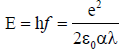(1)

Isolating product Eλ on the left side of this equation, leaving only the set of constants on the right side, then allowed defining this distance based quantum of action from the same set of known electromagnetic constants in reference (, equation (17)), where it was named the electromagnetic intensity constant: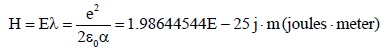(2)

Dividing this constant by the speed of light (c), we then have the surprise of obtaining Planck's time based quantum of action from the same set of electromagnetic constants, which reveals that H=hc directly relates Planck's constant to electromagnetism: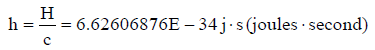(3)

Incidentally, we indeed observe that combining equations (2) and (3) allows defining Planck's time based quantum of action from the same set of electromagnetic constants: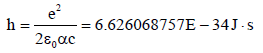(4)

Close analysis shows that Planck's quantum of action is time based only due to the fact that it is equal to energy corresponding to 1 orbit that an electron would run about a hydrogen atom nucleus if it was free to so translate at the mean distance from the nucleus at which the psifunction averages out for the rest orbital of a hydrogen atom.

It was Louis de Broglie who discovered this relation as he observed that Planck's constant was exactly equal to the product of the electron Bohr orbit momentum by the length of the Bohr orbit, an orbit whose radius exactly matches the mean distance that which the probabilistic density of the psi function reaches maximum for the hydrogen atom ground state. Since the hydrogen rest orbital resonance state is key to establishing all other electronic orbital resonance states, this explains why Planck's quantum of action based QM provides so precise information about electronic orbitals: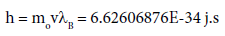(5)

Strangely, this precise Bohr atom electron momentum based definition of Planck's constant discovered by de Broglie is nowhere to be found in the formal literature, nor is any definition correlated to electromagnetic constants, neither at NIST nor in the CRC Handbook of Chemistry & Physics .

Even the obvious definition of h from equation (4) obtained from the known electromagnetic constants set derived from equation (1) is also nowhere to be found, which implies that h seems to still considered a measured constant, not a derived constant.

Since the Bohr orbit is λB=3.32491846E-10 meter long, the total amount of translational energy induced at the Bohr orbit can be obtained by multiplying Planck's quantum of action by the number of times this distance needs to be traveled in 1 second at the Bohr orbit classical velocity (v=2187691.253 m/s) for the total amount of the Bohr ground state energy to be accounted for (h multiplied by v/λB) which is why Planck's constant is related to time: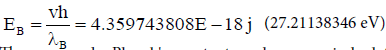(6)

The reason why Planck's constant can be so precisely defined from the non-relativistic velocity calculated for the Bohr radius is precisely because the Bohr radius is obtained from the Coulomb equation, which allows calculating the correct amount of adiabatic energy induced at the real mean hydrogen atom rest orbital, thus the correct amount of electromagnetic energy corresponding to one orbital cycle.

The outcome is that dividing an amount of electromagnetic energy by Planck's constant provides the exact electromagnetic frequency of this amount of energy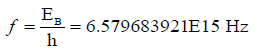(7)

and dividing the speed of light (c) by this electromagnetic frequency provides the electromagnetic wavelength of this amount of energy: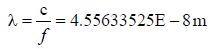(8)

which is the established procedure for calculating electromagnetic wavelength and frequency of energy quanta.

But from equation (2), dividing the electromagnetic intensity constant by the amount of energy induced at the Bohr orbit also provides the same absolute wavelength: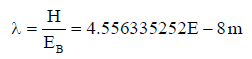(9)

Consequently, the Bohr ground state energy can be obtained from the distance based quantum of action and the absolute wavelength of the carrying energy induced at the Bohr orbit: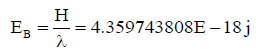(10)

Which disconnects fundamental energy calculation from any need to use the Bohr atom ground state orbit parameters, and rather relates it to electromagnetic parameters, and shows that energy calculation can be disconnected from the flow of time.

#### Separating the Carrying Energy of a Particle from the Energy of Its Rest Mass

One interesting outcome of the new definition of energy revealed by equation (1) is that it allows in ref.  to define local electric and magnetic fields to represent the energy of localized individual photons with the wavelength of an electromagnetic photon as the only variable, all other parameters being the well known set of electromagnetic constants: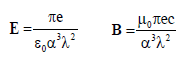(11)

Interestingly, the same equations can directly represent the electric and magnetic fields of the rest mass energy of an electron by using the electron Compton wavelength: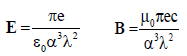(12)

Having established in references [7,20] that the carrying energy of a particle such as the electron has the same electromagnetic structure as that of a free moving photon, this provided the opportunity to unify equations (11) and (12) to build relativistic field equations for the moving electron having the wavelength of the carrying energy and that of rest mass energy of the particle as the only variables. Simple addition and simplification of the magnetic fields parameters of both carrying energy and rest mass energy of the electron does provide the correct unified equation :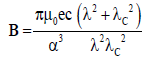(13)

But combining their electric fields turns out to be much more complex, because as mentioned previously, in the trispatial space structure, the charge of electrons is related to momentum in the negative direction along Y-x axis while the electric aspect of its carrying energy can only be momentum presumably due to oscillation on the Y-y/Y-z plane of the electromagnetic half of the carrying energy quantum.

Pending the eventual development of a specific integration procedure that would mathematically resolve this relation in Y-space, the issue can be indirectly resolved by redefining the relativistic velocity parameter v in equation E=vB stemming from the Lorentz force equation, to involve only the wavelengths of the energies of the carrying energy and of the rest mass energy of the particle [20,22]: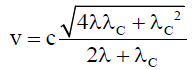(14)

So, by multiplying equation (14) defining the value of v, by relativistic equation (13) defining the value of B, the following relativistic electric fields equation complementary to magnetic fields equation (13) can be obtained for the moving electron :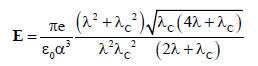(15)

From equations (13) and (15), any relativistic electron velocity can now be calculated from the wavelength of its carrying energy and the rest mass energy wavelength of the electron as the only variables, with the usual equation v=E/B.

#### The Trispatial LC Equation for Permanently Localized Photons in the 3-Spaces Geometry

The next equation is a trispatial LC equation developed in reference  showing the momentum sustaining half of a free moving photon's energy located in X-space as it propels its other half, which is "translationally inertly" oscillating between Y-space and Z-space. Since the only energy that can sustain longitudinal momentum in space is located in X-space, this second half of a photon's energy is translationally inert within Y-spaces and Z-spaces along their x axes: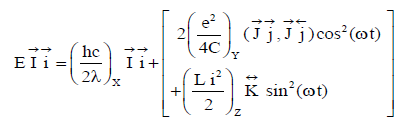(16)

where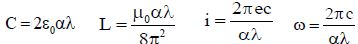(17)

This even split of a photon's energy between an amount of momentum sustaining energy, propelling an equal amount of electromagnetic energy transversely oscillating within two perpendicularly oriented mutually orthogonal 3D spaces, is what explains in this space geometry why the speed of light can only be constant in vacuum .

For simplicity, this oscillating structure allows observing that the two half-photons of Louis de Broglie's hypothesis (two electric charges) are shown as oscillating along the Y-y axis. Given that in the case of a photon, no motion is possible along the perpendicular Y-x axis in this space geometry, this provides a possible explanation to the observed null value of the electric charges presumed to exist in electromagnetic photons in de Broglie's hypothesis, since that in this space geometry the minus sign of the electron charge is related to momentum sustaining energy being oriented in the negative direction along the Y-x axis, while the positive sign of the positron charge is related to the momentum sustaining energy being oriented in the positive direction along this axis. This will be made more obvious with equations (20) and (21) that define the trispatial LC equations of electrons and positrons.

Replacing the inductance and capacitance representations by their equivalent electric and magnetic fields representations shown as equations (11) allows observing them oscillating from one state to the other within the Y-space/Z-space complex in the trispatial space geometry in relation with the momentum sustaining energy of the particle in normal X-space: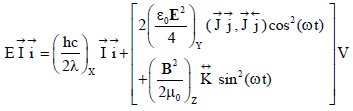(18)

Where V is the related theoretical stationary isotropic volume that the incompressible oscillating kinetic energy quantum would occupy if it was immobilized as a sphere of isotropic density, as defined in ref. :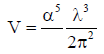(19)

#### The Trispatial LC Equations Describing the Rest Masses of the Electron and the Positron

The trispatial LC equations derived in reference , describe the inner circulation of the energy constituting the invariant rest masses of the electron and positron after the decoupling of the 1.022 MeV mother photon. The trispatial LC equation for the electron is thus: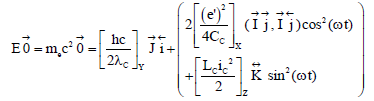(20)

And for the invariant rest mass of a positron: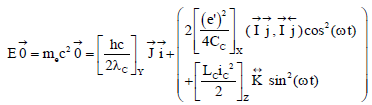(21)

where λc is the electron Compton wavelength.

These representations allow observing that the electric momentum sustaining half of the particle's energy located in Y-space is oriented in the negative direction along the Y-z axis for the electron and in the positive direction for the positron. We can also observe that no energy remains to induce any momentum along the X-x axis of normal space since the energy now oscillating between Z-space and X-space can now only oscillate on the X-y/X-z plane due the constraints of the decoupling process , which is oriented perpendicularly to axis X-x, which is the only direction that allows momentum to be expressed as a velocity in plane wave treatment in the trispatial geometry. This oscillation is represented here as being aligned along the X-y axis.

#### The Trispatial LC Equations Describing a Moving Electron in the Trispatial Geometry

Equations (13) and (15) previously established the inner structure of the relativistic electric and magnetic fields of a moving electron, whose velocity can then be calculated with equation v=E/B.

In Table 1 equations (16) and (20) are used to provide a trispatial LC representation of the same electron moving at relativistic velocity, by using the fields representations of equations (11) for the carrying energy and the fields representation of equation (12) for the rest mass energy of the electron. It can be observed that only possible momentum sustaining energy has to be located in normal X-space along X-x axis.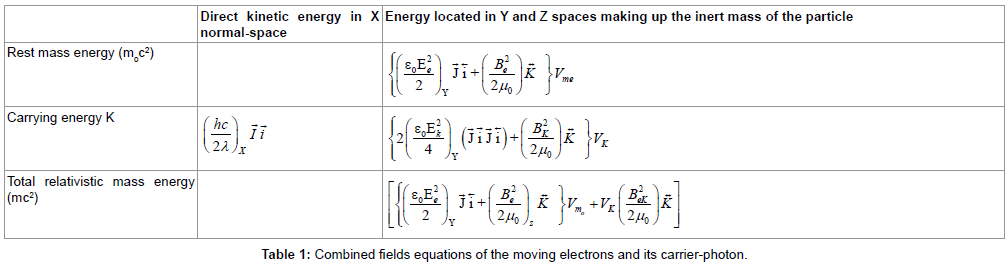Pending the potential development some more advanced integration means to unify further these equations, this table seems to be the next best unifying presentation of the electron in motion in the trispatial space complex.

#### The Last Challenge

Now that moving electromagnetic photons and massive electrons and positrons have been described in the trispatial space geometry, time has come to address the issue of the last two remaining members of the stable set, the up and down quarks, which are the only charged and massive scatterable elementary components of all atomic nuclei, and that up to now have not been linked to the series of kinetic energy transformation processes that unites the other members of the set.

Since up and down quarks "live" in the nuclei of atoms, quantum of action constants h and H that are quite appropriate to calculate the momentum sustaining energy of elementary particles, are not appropriate to deal with energy induction, since this energy is induced as a function of the inverse square of the distance separating any two electrically charged particles, which implies use a radial, or axial, distance with respect to the wavelength, even with their proper definitions from the known electromagnetic constants set (ref: equations (2) and (4)).

As observed with equation (6), calculation of the Bohr ground state energy makes no direct reference to the distance between the electron and the nucleus, and calculates the correct amount of energy strictly from orbital considerations that are fundamentally perpendicular to the direction of energy induction.

What is required is a constant acting axially, that is, perpendicularly to the plane on which the translational motion of an electron can be expressed, which is representable by the Hamiltonian.

Such an appropriate energy induction constant can be defined from the Coulomb equation, since this equation effectively calculates the energy induced at the Bohr orbit as a function of the inverse square of the actual distance separating the Bohr orbit from the central proton. We can thus write that at distance rB the energy induced will be: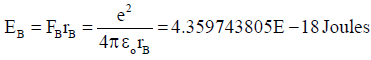(22)

which matches the energy calculated with equation (6) from orbital considerations and with equation (10) from electromagnetic considerations.

This precise quantity of kinetic energy is permanently and adiabatically induced at the mean hydrogen rest orbital  and does not depend on the time elapsed as previously highlighted. The only possible way for this amount of energy to vary is for the distance between the electron and the proton to vary.

The required electrostatic energy induction constant, that we will name K and that could be seen as the "quantum of induction", can be established in two different manners, the fist method stems from the analysis of the manner in which a photon of energy 1.022 MeV or more can decouple into a pair of electron-positron in the 3-spaces geometry as established in reference , and the second method consists in simply multiplying equation (22) by rB squared: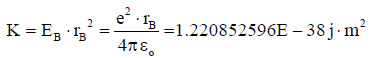(23)

With this constant, it is possible to enter the hydrogen nucleus "vertically" or "axially", so to speak, by varying the distance r between two charged particles in equation E=K/r2, and so establish the exact amounts of adiabatic energy induced in each of the inner components of the proton and the neutron (Table 2), thus allowing us to finally establish coherent trispatial LC equations for the up and down quarks and their carrier-photons, as analyzed in reference .

Table of the energies contained in the effective masses of quarks Up and Down, estimated on the assumptions that unit charge would be a measure of decoupling distance of electron/positron pairs in electrostatic space
Particle r'=a0α E=K/r2 λ=hc/E
Electron r'e=3.861592641E-13 m 0.5109989027 MeV 2.426310215E-12 m
Quark up r'eu=2.574395094E-13 m 1.149747531 MeV 1.078360096E-12 m
Quark down r'ed=1.287197547E-13 m 4.598990173 MeV 2.69590021E-12 m

Table 2: Calculated effective rest mass energies of up and down quarks.

Indeed, dealing with axial energy induction in atomic structures seems to be the only way that such a space geometry can be explored, which induces acute awareness of the adiabatic levels of energy permanently induced in all massive particles making up massive objects and which is not representable by the Hamiltonian when translational motion, thus momentum, is prevented from being expressed by the translationally immobilizing electromagnetic equilibrium states that they generally are captive in. The issue of axial adiabatic energy induction in atoms is analyzed in reference .

This analysis highlighted the surprising fact that although the physics community has been aware since Coulomb that energy is induced as a function of the inverse square of the distance between charged particles, and since the beginning of the 20th century that charged particles organize axially in atomic structure, Classical Mechanics, Relativistic Mechanics, Quantum Electrodynamics, Electromagnetic Theory and Quantum mechanics all apparently still deal with energy "horizontally" so to speak, as witnessed by the fact that the Hamiltonian, basic to quantum physics, and stemming from a reformulation of Classical Mechanics, can fundamentally represent energy only if it involves the momentum of a "moving" particle, which causes it to be unable, for example, to represent the adiabatic amount of 27.2 eV energy induced at the hydrogen ground state if the electron is translationally immobilized by the local electromagnetic equilibrium state.

Clear awareness of adiabatically stabilized energy in atomic structures also sheds new light on gravitation and on how the collected data on spacecrafts' hyperbolic trajectories, systematic so-called anomalous spacecrafts' flybys accelerations, and systematic so-called anomalous rotation slowdown of all spacecrafts, can be interpreted [9,10,25-28].

#### The Fractional Charges of Up and Down Quarks

In Y-space, the sign intensity of the positive and negative electric charges of electrons and positrons is tied to the distance from the trispatial junction-point in Y-space (r' in Table 2) at which their electric energy expresses their momentum in opposite directions parallel to the Y-x axis. The diminished charges of the up and down quark are thus related in the trispatial geometry to the precise shorter distances that the stress of their equilibrium states forces them to express their momentum in opposite directions parallel to this axis within the structure of nucleons  See parameter r' shown in Table 2.

In the trispatial geometry, momentum that cannot be expressed as a "velocity", is expressed as a "measurable pressure" in the direction of application of the Coulomb force in X-space in the case of the least action electromagnetic equilibrium states , and is expressed as a "measurable intensity" of the electric charge of a particle in Y-space [7,23]. Their related increased rest masses are similarly related to these shorter distances as a function of the previously mentioned axial inverse square law of distance from the trispatial junctions  shown in Table 3.

Up quark Down quark
Rotation diameter r=r’sin 600=3.344237326E-13 m
Rotation radii 2r/3=2.229491551E-13 m r/3=1.114745775E-13 m
Orbit lengths D=2πr 1.400830855E-12 m 7.004154277E-13 m
Quark masses in kg m=E•1.6E-19/c2 2.049610923E-30 kg 8.198443779E-30 kg

Table 3: Relation between up and down quarks masses and their translational and rotational radii about the Y-z axis and the X-x axis in the trispatial geometry.

#### The Trispatial LC Equations of the Up and Down Quarks

The outcome of this axial exploration of the inner structure of nucleons came in support of the possibility that up and down quarks could simply be positrons and electrons whose masses and charge characteristics would be warped into these altered states by the stresses imposed by these most energetic least action equilibrium states that electrons and positrons can reach in nature [4,23].

The trispatial LC equation for the up quarks is: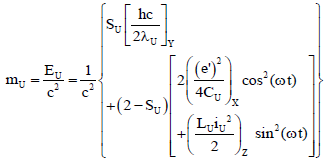(24)

where λu is the wavelength of the energy making up the invariant rest mass of the up quark, and Su is the up quark magnetic drift stress constant , with dimensionless value 2/3.

And the trispatial LC equation for the down quark is:(25)

Where λd is the wavelength of the energy making up the invariant rest mass of the down quark, and Sd is the down quark magnetic drift stress constant , with dimensionless value 1/3.

In both cases, the trispatial LC equations describing the carrying energy of up and down quarks are identical to equation (16) for the permanently localized photon.

Since the three quarks of a proton (uud) as well as those of a neutron (udd) simultaneously translate about two different axes in the tri-spatial geometry , that is the coplanar axis Y-z and the normal space X-x axis, there would be need to build 6 tables such as Table 1 to represent each possible configuration of the three quarks whose motion about coplanar Y-z axis would be sustained by their carrierphotons as perceived from X-space, each possessing an energy of approximately 310 MeV , Table III), and thee more such Tables to represent each carrier-photon being considered the propelled particle as perceived from Y-space, being propelled by the quarks then acting as their carrier-photons, sustaining their motion about the X-x axis.

Obviously, this set of trispatial LC equations is only an entry step into this space geometry, considering that they seem to already have reached their representational limit with these table representations.

#### Conclusion

These equations summarize the description of all stable and massive point-like behaving electromagnetic particles that have been experimentally detected at the sub-microscopic level. The trispatial LC descriptions of electron, muon and tau particles before they release momentary excess mass in the form of neutrinos are derived in reference .

Similar trispatial LC equations can of course be defined for all pointlike behaving scatterable electromagnetic sub-components of all unstable partons that were detected, but their description exceeds the scope of the present paper, and are not required to describe normal matter, since they exist only fleetingly and amount to practically nothing in the universe since their short life span prevents any accumulation of these particles.

These conclusions from the analysis of the manner in which electromagnetic energy is likely to behave in this expanded space geometry are tentative entry level in many respects and may require some re-focusing to better formulations, and may even be overly speculative pending experimental confirmation, which means that deep formal analysis remains to be carried out.

But complete mathematization of axially induced adiabatic energy in atomic structures, and whose existence becomes so obvious in the 3-spaces model, could bring to fruition some important applied physics benefits hinted at in reference , that will remain out of reach until such mathematization has been accomplished.

So after having thoroughly explored the momentum based "translational plane" of particle physics, so to speak, mainly by means of the Hamiltonian, the last challenge of modern physics may really be to finally go 3 dimensional to finally integrate the so promising orthogonally oriented adiabatic energy induction process.

#### References

Select your language of interest to view the total content in your interested language

### Article Usage

• Total views: 1489
• [From(publication date):
March-2017 - Dec 10, 2019]
• Breakdown by view type
• HTML page views : 1343Can't read the image? click here to refresh